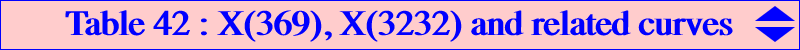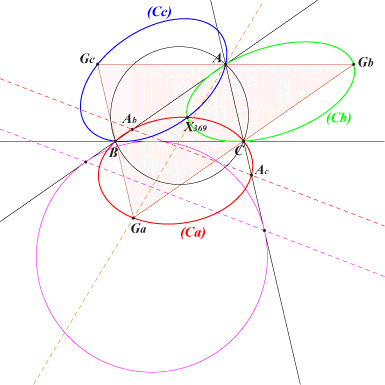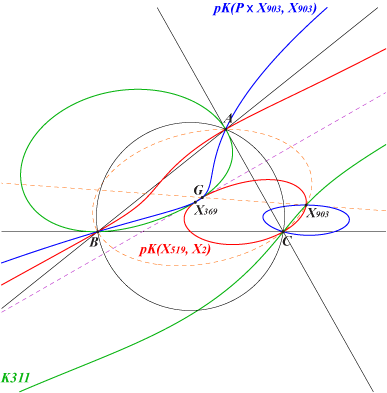There exist points A', B', C' on segments BC, CA, AB, respectively, such that AB' + AC' = BC' + BA' = CA' + CB' = (a + b + c)/3, and the lines AA', BB', CC' concur in X(369) called the first trisected perimeter point in CTTC and ETC. Similarly, there exist points A', B', C' on segments BC, CA, AB, respectively, such that B'C + C'B = C'A + A'C = A'B + B'A = (a + b + c)/3, and the lines AA', BB', CC' concur in X(3232) called the second trisected perimeter point in ETC. These two points X(369) and X(3232) are isotomic conjugates.Conics Three conics passing through X(369) and three conics passing through X(3232) Nikolaos Dergiades (Hyacinthos #17498) has found three conics that contain this point X(369). (Ca) has equation : (a + b + c) x^2 + (a + b - 2 c) x y + (a + c - 2 b) x z + (a - 2 b - 2 c) y z = 0, the other conics (Cb) and (Cc) similarly.(Ca) passes through B, C, Ga (A-vertex of the antimedial triangle), Ab, Ac. These two latter points are the intersections of the sidelines AB, AC with the homothetic under h(A, 2/3) of the line passing through the contacts of the A-excircle with these same sidelines. These three conics generate a net of conics passing through X(369) and two other points Z1, Z2 which are always imaginary. Each conic (C) of the net can be written under the form : (C) = p (Ca) + q (Cb) + r (Cc), where (p : q : r) is any point of the triangle plane.Naturally, the isotomic transforms (C'a), (C'b), (C'c) of these three conics must contain X(3232). (C'a) has equation : (a + b + c) y z + (a + b - 2 c) x z + (a + c - 2 b) x y + (a - 2 b - 2 c) x^2 = 0.Cubics The union of a line (Da) passing through A and (Ca) is a decomposed circum-cubic (Ka) that contains X(369) and Z1, Z2. (Kb) and (Kc) being defined likewise, any cubic of the form (K) = (Ka) + (Kb) + (Kc) contains X(369) and Z1, Z2. Three pivotal cubics obtained with cevian lines When (Da), (Db), (Dc) are the cevian lines of a point Q, (K) is a circum-cubic passing through X(903), the isotomic conjugate of the infinite point X(519) of the line IG.In this case, (K) becomes a pivotal cubic if and only if Q is : • G giving the cubic K1148 = pK(X519, X2), • X(8) giving the cubic K311 = pK(X2, X320), • the midpoint X(3679) of G-X(8) giving the cubic K1147 = pK(X4945, X903).   The isotomic transforms of these three pKs are three pKs that contain X(3232). In particular, X(3232) lies on K311 since it is self-isotomic and on pK(X903, X903), the isotomic transform of K1148.Other pivotal cubics A pivotal cubic pK(Ω, P) passing through X(369), Z1, Z2 always contains X(519) and must have • its pole Ω on K1149 = pK(X4908, X2), • its pivot P on K311 = pK(X2, X320), • its isopivot P* on K1150 = pK(X4908, X8). See also K1151.Quartics The decomposed quartic which is the union of pK(X519, X2) and the line at infinity and the Parry quartic Q045 generate a pencil of circum-quartics which contain X(369). Properties of these quartics : • the tangents at A, B, C concur at a point X lying on the curve and on the line X(1)-X(2). • each quartic Q contains X(2), X(519), the midpoints of ABC, the infinite points of the sidelines of ABC. • it follows that Q has three real asymptotes parallel to the sidelines of ABC. • Q has a fourth real asymptote which is parallel to the line X(1)X(2). This asymptote is the same for all undecomposed quartics.Other curves X(369) lies on the Dergiades septic Q100.